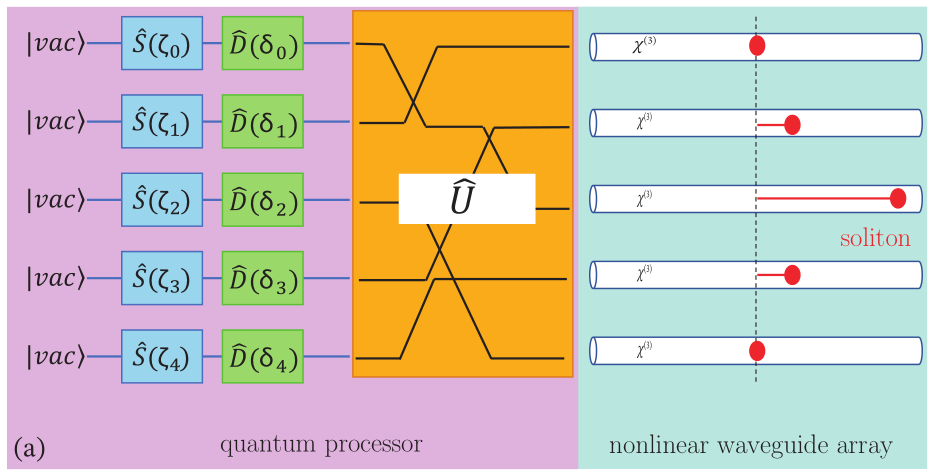# Boson sampling solitons by quantum machine learninghttps://arxiv.org/abs/2110.12379

We use a neural network variational ansatz to compute Gaussian quantum discrete solitons in an array of waveguides described by the quantum discrete nonlinear Schroedinger equation. By training the quantum machine learning model in the phase space, we find different quantum soliton solutions varying the number of particles and interaction strength. The use of Gaussian states enables measuring the degree of entanglement and the boson sampling patterns. We compute the probability of generating different particle pairs when varying the soliton features and unveil that bound states of discrete solitons emit correlated pairs of photons. These results may have a role in boson sampling experiments with nonlinear systems and in developing quantum processors to generate entangled many-photon nonlinear states.